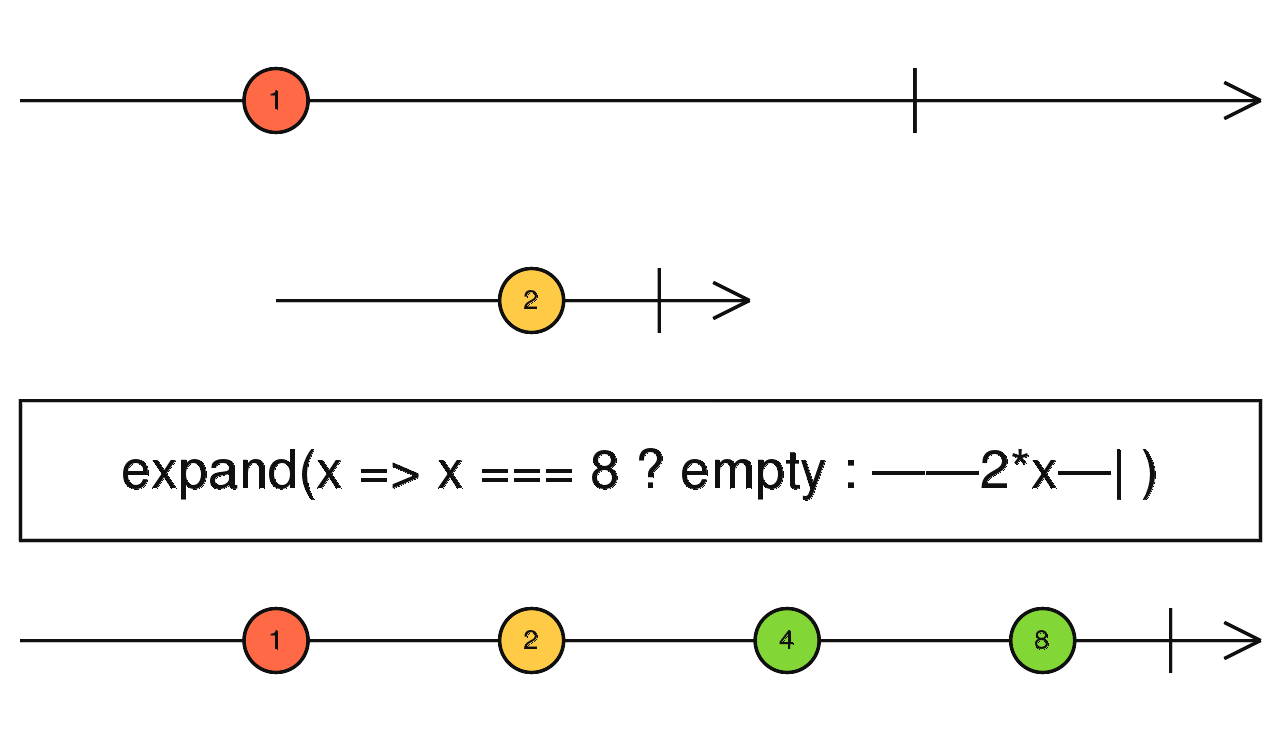/RxJS

# expand

`function` `stable`

Recursively projects each source value to an Observable which is merged in the output Observable.

### `expand<T, R>(project: (value: T, index: number) => ObservableInput<R>, concurrent: number = Number.POSITIVE_INFINITY, scheduler: SchedulerLike = undefined): OperatorFunction<T, R>`

#### Parameters

 project A function that, when applied to an item emitted by the source or the output Observable, returns an Observable. concurrent Optional. Default is `Number.POSITIVE_INFINITY`. Maximum number of input Observables being subscribed to concurrently. scheduler Optional. Default is `undefined`. The `SchedulerLike` to use for subscribing to each projected inner Observable.

#### Returns

`OperatorFunction<T, R>`: An Observable that emits the source values and also result of applying the projection function to each value emitted on the output Observable and and merging the results of the Observables obtained from this transformation.

## Description

It's similar to `mergeMap`, but applies the projection function to every source value as well as every output value. It's recursive.Returns an Observable that emits items based on applying a function that you supply to each item emitted by the source Observable, where that function returns an Observable, and then merging those resulting Observables and emitting the results of this merger. Expand will re-emit on the output Observable every source value. Then, each output value is given to the `project` function which returns an inner Observable to be merged on the output Observable. Those output values resulting from the projection are also given to the `project` function to produce new output values. This is how expand behaves recursively.

## Example

Start emitting the powers of two on every click, at most 10 of them

```import { fromEvent, of } from 'rxjs';
import { expand, mapTo, delay, take } from 'rxjs/operators';

const clicks = fromEvent(document, 'click');
const powersOfTwo = clicks.pipe(
mapTo(1),
expand(x => of(2 * x).pipe(delay(1000))),
take(10),
);
powersOfTwo.subscribe(x => console.log(x));```